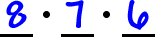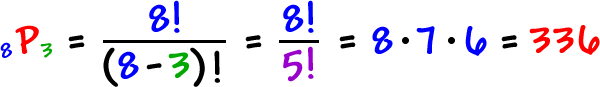* For this one, order does NOT matter!

We did this problem before:

If we have 8 books, how many ways can we arrange 3 on a
bookshelf?

We figured it out with slots:But, using the formula gave us the same thing:Here's a different question for you:

If we have 8 books and we want to take 3 on vacation with us, how
many ways can we do it?

What's the difference between these problems?

ORDER DOESN'T MATTER!

In the first problem, we were arranging the 3 books on a shelf...  and in the second problem, we're just tossing the 3 books in a suitcase.

So, if order doesn't matter, we'll just divide it out!

Arranging the 3 books is 3!